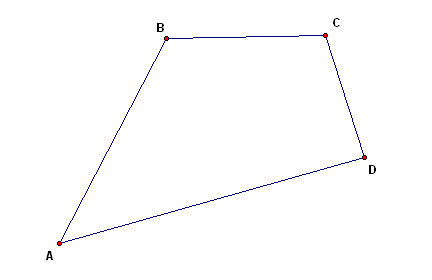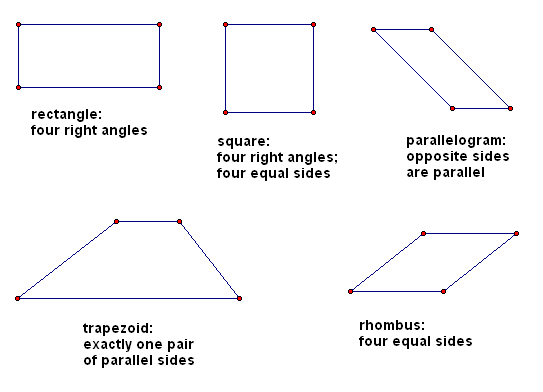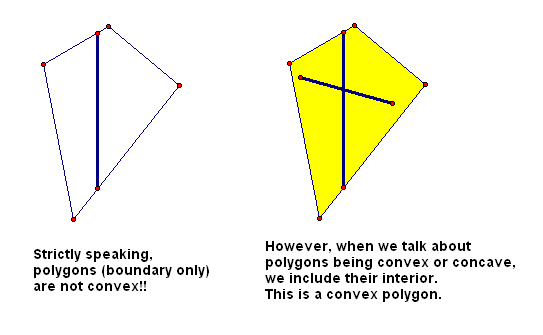Recall from Introduction to Polygons that a polygon is a closed figure in a plane made by joining line segments,
where each line segment intersects exactly two others.

In this section, we continue our exploration of four-sided polygons:

A quadrilateral is a polygon with four sides.

Sides, angles, and vertices that are next to each other in a polygon are called consecutive.
For example, in the quadrilateral below:

• $\,\overline{AB}\,$ and $\,\overline{BC}\,$ are consecutive sides
(Note that consecutive sides intersect at a single point.)
• $\,\angle C\,$ and $\,\angle D\,$ are consecutive angles
• $\,A\,$ and $\,D\,$ are consecutive verticesWhen a quadrilateral is named, recall that the vertices are always listed in a consecutive order.
Therefore, the quadrilateral above could be named $\,ABCD\,$ or $\,BCDA\,$, but not $\,ACBD\,$.

In a polygon, objects that are not consecutive are said to be nonconsecutive.
In a quadrilateral, nonconsecutive objects are given a special name—they are said to be opposite.
For example, in the quadrilateral above:

• $\,\overline{AD}\,$ and $\,\overline{BC}\,$ are opposite sides
• $\,\angle B\,$ and $\,\angle D\,$ are opposite angles
• $\,A\,$ and $\,C\,$ are opposite vertices

DEFINITION diagonal
A diagonal of a polygon is a line segment that connects any two nonconsecutive vertices.

A diagonal of a quadrilateral separates the quadrilateral into two triangles, as shown below:By using the fact that the angles in a triangle sum to 180°, we thus have the following:

THEOREM
The sum of the interior angles in a quadrilateral is 360°.

It follows immediately that if a quadrilateral has four equal angles, then they must each be 90°.

There are several important types of quadrilaterals, which are classified according to extra properties that their angles or sides possess:DEFINITION rectangle
A rectangle is a quadrilateral with four right angles.
DEFINITION square
A square is a quadrilateral with four right angles and four equal sides.
DEFINITION parallelogram
A parallelogram is a quadrilateral whose opposite sides are parallel.
DEFINITION trapezoid
A trapezoid is a quadrilateral that has exactly one pair of parallel sides.
The parallel sides are called the bases of the trapezoid.
The non-parallel sides are called the legs of the trapezoid.
DEFINITION rhombus
A rhombus is a quadrilateral with four equal sides.

Note: You can use either rhombuses or rhombi for the plural of rhombus.

It is easy to prove (later on) that every square, rectangle, and rhombus must be a parallelogram.

CONVEX/CONCAVE POLYGONS

Consider two oddly-shaped fields, shown below:If you pick any two points in the first field, and walk in a straight line from one point to the other, then you'll remain inside the field.
Geometric figures with this property are said to be convex.

However, there are points in the second field with the property that the straight-line path between them takes you outside of the field.
Geometric figures with this property are said to be concave.
(Memory device: it is caved in)

DEFINITION convex, concave
A set of points is convex   if and only if    given any two points in the set, the line segment containing the two points lies entirely in the set.
A set of points that is not convex is concave.

Recall that, strictly speaking, a polygon does not include its interior—the polygon is only the "boundary".
So, strictly speaking, line segments connecting points on the polygon go outside the polygon (first picture below).

However, when we talk about polygons being convex or concave, it is conventional to include the interior.Squares, rectangles, parallelograms, trapezoids and rhombuses are all convex polygons.

Master the ideas from this section